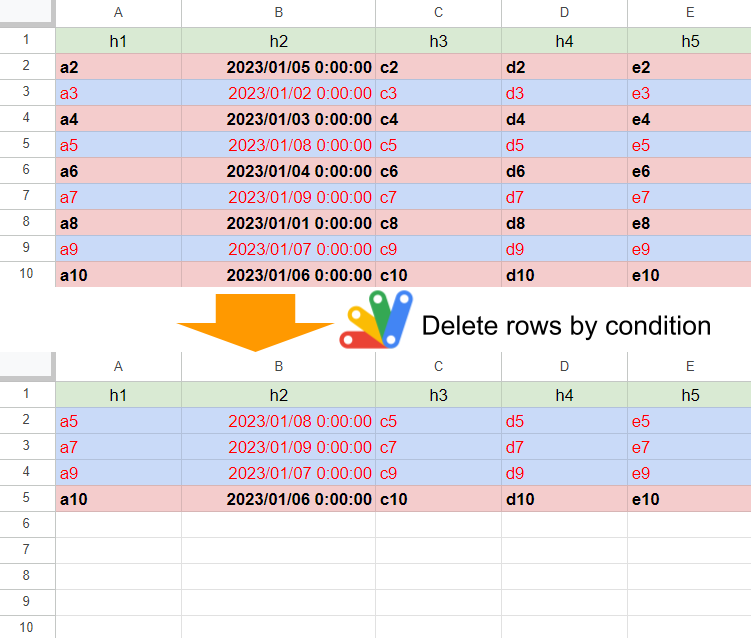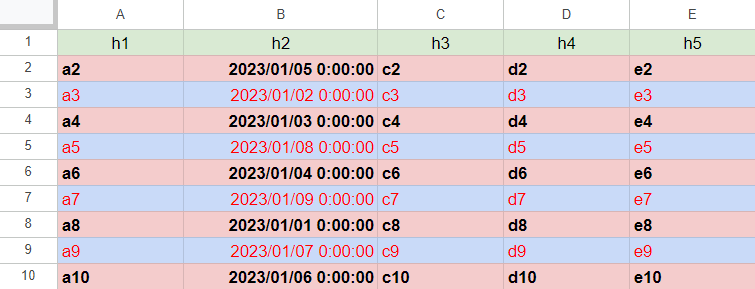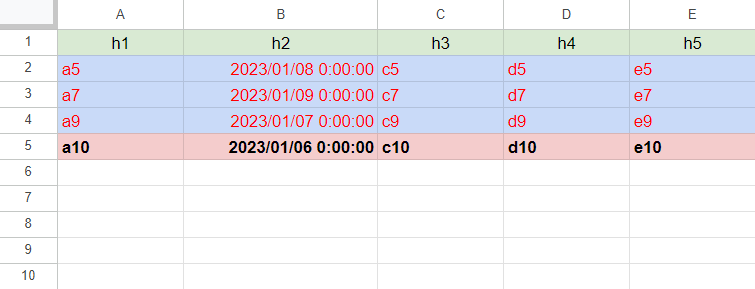Gists## Description

In this report, I would like to introduce a sample script for efficiently deleting rows by conditions on Google Spreadsheet using Google Apps Script. Recently, I had a situation for being required to achieve this situation. In my report, it has already known that when Sheets API is used, the rows can be efficiently deleted by a condition. Ref However, in that case, Sheets API couldn’t be used. Under this situation, I came up with a method. In this report, I would like to introduce this method.

First, I would like to show you the sample Spreadsheet as follows.In this sample Spreadsheet, there are 5 columns. And, column “B” has the date object randomly put. Those data values are 2023/01/01 00:00:00 to 2023/01/10 00:00:00 and all values are unique values in this column. Under this condition, it was required to delete all rows before 2023/01/05 (this value is a sample condition for the above Spreadsheet.) with the low process cost and keeping the background colors, the text styles, and the text alignments. The output situation is as follows.In the actual situation, the number of rows was about 100,000. I thought that the following 3 patterns.

## Sample 1

function sample1() {
const threshouldDate = new Date("2023-01-05T06:00:00").getTime();
const range = sheet.getDataRange();
const { v, c, s, av, ah } = values.reduce((o, r, i) => {
if (r.getTime() >= threshouldDate) {
o.v.push(r);
o.c.push(valueColors[i]);
o.s.push(valueStyles[i]);
o.av.push(valueVAligns[i]);
o.ah.push(valueHAligns[i]);
}
return o;
range.clear().offset(0, 0, v.length, v.length).setValues(v).setBackgrounds(c).setTextStyles(s).setVerticalAlignments(av).setHorizontalAlignments(ah);
}


When this script is run to the above sample Spreadsheet, the above-expected result is obtained. When the number of rows is 100,000 and 50,000 rows are required to be deleted from these rows, the average process time was 67 seconds.

## Sample 2

function sample2() {
const threshouldDate = new Date("2023-01-05T06:00:00").getTime();
const range = sheet.getDataRange();
const [, ...values] = range.getValues();
values.reverse().forEach((r, i, a) => {
if (r.getTime() <= threshouldDate) {
sheet.deleteRow(a.length - i + 1);
}
});
}


When this script is run to the above sample Spreadsheet, the above-expected result is obtained. When the number of rows is 100,000 and 50,000 rows are required to be deleted from these rows, an error of “Exceeded maximum execution time” occurs.

## Sample 3

function sample3() {
const threshouldDate = new Date("2023-01-05T06:00:00").getTime();
const range = sheet.getDataRange();
const dstValues = [header, ...values.map(r => r.getTime() > threshouldDate ? r : header)];
range.setValues(dstValues).removeDuplicates(); // or range.setValues(dstValues).removeDuplicates();
}


When this script is run to the above sample Spreadsheet, the above-expected result is obtained. When the number of rows is 100,000 and 50,000 rows are required to be deleted from these rows, the average process time was 13 seconds.

## Summary

In this report, I introduced the method for efficiently deleting rows by conditions on Google Spreadsheet using Google Apps Script. From this report, I obtained the following result.

Pattens Process time [s]
Sample 1: After the values, backgrounds, text styles, and text alignments were retrieved, remove the row values in the arrays by a condition, and overwrite the cells. 67
Sample 2: Rows are deleted with 'deleteRow' method. Exceeded maximum execution time (over 360 seconds)
Sample 3: After the rows for deleting were overwritten by the header row, rows are deleted with 'removeDuplicates' method. 13

As a result, it was found that when the rows are deleted with keeping the background colors, the text styles, and the text alignments, sample pattern 3 using “removeDuplicates” is suitable. But, if this method is used for other situations, I think that it is required to check whether the duplicate rows are existing before the rows are deleted. So, I think that the above script of “Sample 3” should be modified as follows.

const check_ = values => [...values.reduce((m, e) => {
const k = e.join("");
return m.set(k, m.has(k) ? true : false);
}, new Map()).values()].includes(true);

function modified_sample3() {
const threshouldDate = new Date("2023-01-05T06:00:00").getTime();
const range = sheet.getDataRange();
console.log("This method cannot be used.")
return;
}
const dstValues = [header, ...values.map(r => r.getTime() > threshouldDate ? r : header)];
range.setValues(dstValues).removeDuplicates(); // or range.setValues(dstValues).removeDuplicates();
}


## Principle of this method

Explain the principle of this method as follows. The method of removeDuplicates() delete rows the duplicated rows. In that case, the 1st row of the duplicated rows is left. This method uses this. When you see the Spreadsheet before the script is run, you can see both the header row and the data rows. In this method, all the duplicated rows are replaced with the header row. Under this condition, when removeDuplicates() is run, the header row is left while the duplicated rows of data rows are deleted.

For example, when the header row is not existing, first, please insert the temporal header row into the 1st row, and run this script. And, please delete the temporal header row.

The process cost of this method depends on the number of cells. When the number of cells is large, the process cost becomes high.

Also, when the number of cells is large, the process costs of getValues and setValues become high. Ref and Ref As a test, I checked the following 2 patterns using the above “sample3” script.

1. 100,000 rows and 26 columns: 130 seconds

2. 100,000 rows and 5 columns: 90 seconds

When I tested this, the process cost of “removeDuplicates” was about 10 % of all costs of “sample3”. And, the process cost of getValues and setValues is about 85 % of all costs. It is found that the process cost for transferring data from Spreadsheet is very high. For example, when getValues and setValues are modified to Sheets API, “100,000 rows and 5 columns: 90 seconds” is changed to 30 seconds.

As additional information, in this case, the process costs of the scripts “sample1” and “sample2” are much higher than that of “sample3”.

From this test,

1. Process cost for deleting rows while keeping the cell format depends on the number of cells.
• My proposed script supposes the cell format that is not patterned, either. For example, that is the situation in which the cell backgrounds are randomly set.
2. When the number of rows is large, replacing getValues and setValues with Sheets API will be lower cost.
3. Process cost of “removeDuplicates” is much lower than that of the data transfer to a Spreadsheet.